# insertShape

Insert shapes in image or video

## Syntax

``RGB = insertShape(I,shape,position)``
``RGB = insertShape(___,Name=Value)``

## Description

example

````RGB = insertShape(I,shape,position)` insert the specified `shape` into the truecolor or grayscale image `I`. The function draws the shapes by overwriting pixel values, and returns a truecolor image.```
````RGB = insertShape(___,Name=Value)` specifies options using one or more name-value arguments in addition to the input arguments from the input arguments from the previous syntax. For example, `LineWidth=5` sets the line width value to `5` of the inserted shapes.```

## Examples

collapse all

Read an image into the workspace.

`I = imread("peppers.png");`

Place a circle on the image with a border line width of 5 pixels.

`RGB = insertShape(I,"circle",[150 280 35],LineWidth=5);`

Place a filled triangle and a filled hexagon on the image.

```pos_triangle = [183 297 302 250 316 297]; pos_hexagon = [340 163 305 186 303 257 334 294 362 255 361 191]; RGB = insertShape(RGB,"filled-polygon",{pos_triangle pos_hexagon}, ... ShapeColor=["white","green"],Opacity=0.7);```

Display the resulting image.

`imshow(RGB)`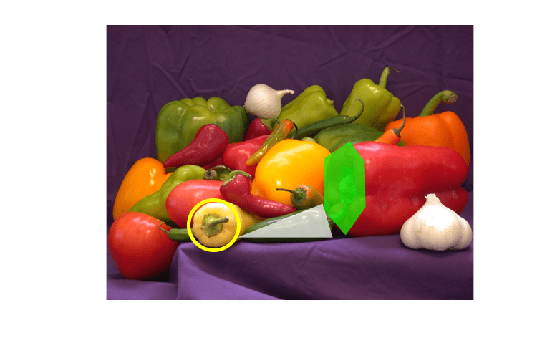## Input Arguments

collapse all

Input image, specified as an M-by-N-by-3 truecolor or an M-by-N grayscale image.

Data Types: `single` | `double` | `int16` | `uint8` | `uint16`

Type of shape, specified as `"rectangle"`, `"filled-rectangle"`, `"line"`, `"polygon"`, `"filled-polygon"`, `"circle"`, `"filled-circle"`, or `"projected-cuboid"`.

Data Types: `char` | `string`

Position of shape, specified according to the type of shape, as described in the table.

ShapePositionExample

`rectangle`

`filled-rectangle`

For one or more axis-aligned rectangles or filled rectangles, specify as an M-by-4 numeric matrix, where each row specifies a rectangle of the form $\left[\begin{array}{cccc}x& y& width& height\end{array}\right]$.

• M is the number of axis-aligned rectangles.

• x and `y` specify the upper-left corner of the rectangle.

• w specifies the width of the rectangle, which is its length along the x-axis.

• h specifies the height of the rectangle, which is its length along the y-axis.

`$\left[\begin{array}{cccc}{x}_{1}& {y}_{1}& widt{h}_{1}& heigh{t}_{1}\\ {x}_{2}& {y}_{2}& widt{h}_{2}& heigh{t}_{2}\\ ⋮& ⋮& ⋮& ⋮\\ {x}_{M}& {y}_{M}& widt{h}_{M}& heigh{t}_{M}\end{array}\right]$`For one or more rotated rectangles, specify in spatial coordinates as an M-by-5 numeric matrix, where each row specifies a rotated rectangle of the form [xctr yctr w h yaw].

• M is the number of rotated rectangles.

• xctr and yctr specify the center of the rectangle.

• w specifies the width of the rectangle, which is its length along the x-axis before rotation.

• h specifies the height of the rectangle, which is its length along the y-axis before rotation.

• yaw specifies the rotation angle in degrees. The rotation is clockwise-positive around the center of the rectangle.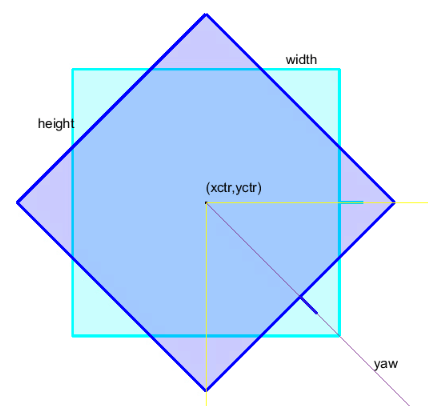`circle`

`filled-circle`

For one or more circles, specify spatial coordinates as an M-by-3 numeric matrix, where each row specifies a circle of the form [xctr yctr radius].

• M is the number of circles.

• xctr and yctr specify the center of the circle.

`$\left[\begin{array}{ccc}xct{r}_{1}& yct{r}_{1}& radiu{s}_{1}\\ xct{r}_{2}& yct{r}_{2}& radiu{s}_{2}\\ ⋮& ⋮& ⋮\\ xct{r}_{M}& yct{r}_{M}& radiu{s}_{M}\end{array}\right]$``line`

For one or more lines, specify spatial coordinates using one of these formats to specify a single line with connected endpoints, multiple lines with the same number of endpoints, or multiple lines with different numbers of endpoints.

• Single lines with connected endpoints — Specify as a P-by-2 matrix, where each row specifies an endpoint in the form [x y], and the endpoint in one row connects directly to the endpoint in the next. P is the number of endpoints in the line.

• Multiple lines with the same number of endpoints — Specify as an M-by-2P matrix, where each row specifies the consecutive endpoints of a single line in the form [x1 y1 x2 y2 ... xp yp]. Each [x y] pair is the coordinates of a single endpoint. M is the number of specified lines, and P is the number of endpoints per line.

• Multiple lines with different numbers of endpoints — Specify as an M-by-1 cell array, where each cell contains the endpoints for a single line as a P-by-2 matrix or a 1-by-2P vector]. M is the number of specified lines, and P is the number of endpoints for each line`polygon`

`filled-polygon`

For one or more polygons, specify in spatial coordinates as an M-by-1 cell array, where each cell contains an L-by-2 matrix of [x y] vertex locations or a 1-by-2L vector of consecutive vertex locations of the form [x1, y1, x2,y2, … xL,yL ].

• M is the number of polygons.

• Each [x y] pair describes a vertex location or endpoint.

• L is the number of vertices in a polygon or endpoints in a line. Each polygon or line can have a different number of vertices or endpoints.` projected-cuboid`

For one or more projected cuboids, specify in spatial coordinates as an 8-by-2-by-M array or an M-by-8 matrix, where M is the number of projected cuboids.

When specified as an 8-by-2-by-M array, each row must contain the [x y] location of a projected cuboid vertex. The vertices connect to form a cuboid with six faces. The order of the input vertices must match the order shown in the diagram.

When specified as an M-by-8 matrix, each row specifies the dimensions of the front-facing and rear-facing sides of a projected cuboid in the form, [x1 y1 w1 h1 x2 y2 w2 h2], where [x1 y1] and [x2 y2] specify the upper-left coordinates of the front-facing and rear-facing sides, respectively, and [w1 h1] and [w2 h2] specify the corresponding widths and heights.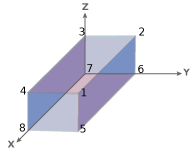Data Types: `single` | `double` | `cell` | `int8` | `int16` | `int32` | `int64` | `uint8` | `uint16` | `uint32` | `uint64`

### Name-Value Arguments

Specify optional pairs of arguments as `Name1=Value1,...,NameN=ValueN`, where `Name` is the argument name and `Value` is the corresponding value. Name-value arguments must appear after other arguments, but the order of the pairs does not matter.

Example: `insertShape(I,"circle",position,Color="yellow")` sets the shape color to yellow.

Before R2021a, use commas to separate each name and value, and enclose `Name` in quotes.

Example: `insertShape(I,"circle",position,"Color","yellow")` sets the shape color to yellow.

Shape border line width, specified as a positive integer, in pixels. This argument applies to only the `"Rectangle"`, `"Line"`, `"Polygon"`, and `"Circle"` shapes.

Data Types: `uint8` | `uint16` | `int16` | `double` | `single`

Shape color, specified as a short color name, color name, vector of color names, three-column matrix of RGB triplets.

The supported colors table lists RGB intensities in the range [0, 1], but you must specify RGB triplets in the range of your selected data type. For example, if specifying this argument as a matrix of `uint8` values, you must convert each intensity value to the range [0, 255]. To convert the listed intensity values to a `uint8` data type, use the code `uint8(255*intensity)`, where intensity is an RGB triplet value listed in the table.

You can specify a different color for each shape or one color for all shapes. To specify one color for all markers, specify `ShapeColor` as a color name or an [R G B] vector.

SpecificationFormatExample
Specify one color for all shapes (or markers)

Short color name or color name

`"r"`

`"red"`

RGB triplet

`[1 0 0]`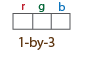Specify a color for each shape (or marker)

Vector of color names

`["red","yellow","blue"]`

Three-column matrix of RGB triplets

```[1 0 0 0 1 1 1 0 1 1 1 1]```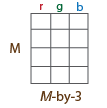This table lists the supported shape colors.

Color NameShort NameRGB TripletAppearance
`"red"``"r"``[1 0 0]``"green"``"g"``[0 1 0]``"blue"``"b"``[0 0 1]``"cyan"` `"c"``[0 1 1]``"magenta"``"m"``[1 0 1]``"yellow"``"y"``[1 1 0]``"black"``"k"``[0 0 0]``"white"``"w"``[1 1 1]`Data Types: `logical` | `uint8` | `uint16` | `int16` | `double` | `single` | `cell`

Opacity of a filled shape, specified as a scalar in the range [0 1]. The `Opacity` argument applies to only the `"filled-rectangle"`, `"filled-polygon"`, and `"filled-circle"` shapes.

Data Types: `double` | `single` | `int8` | `int16` | `int32` | `int64` | `uint8` | `uint16` | `uint32` | `uint64`

Smooth shape edges, specified as a logical `1` (`true`) or `0` (`false`). A `true` value enables an antialiasing filter to smooth shape edges. This value applies to only nonrectangular shapes. When you enable antialiasing requires additional time to draw the shapes.

Data Types: `logical`

## Output Arguments

collapse all

Output image, returned as an M-by-N-by-3 truecolor image.

## Version History

Introduced in R2014a

expand all